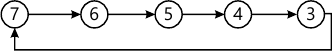MORE IN Digital Electronics
MU Electronics and Telecom Engineering (Semester 3)
Digital Electronics
December 2014
Total marks: --
Total time: --
INSTRUCTIONS
(1) Assume appropriate data and state your reasons
(2) Marks are given to the right of every question
(3) Draw neat diagrams wherever necessary

1 (a) Compare Moore and Mealy machine models.
5 M
1 (b) Design a 4:1 multiplexer using only NAND gates.
5 M
1 (c) Write a VHDL code for full adder.
5 M
1 (d) Convert SR F/F to D F/F.
5 M

2 (a) Implement the following Boolean function with 8: multiplexer F(A,B,C,D)=?m(0,3,5,6,8,9,10,12,14).
10 M
2 (b) State truth table of 3 bit Gray to Binary conversion then design it using 3:8 decoder and additional gates.
10 M

3 (a) Use the quine McCluskey method of minimization and find the expression for the function.
F(A,B,C,D)=?m(0,1,2,3,5,7,8,9,11,14).
10 M
3 (b) Define the following in terms of Logical families
i) Propagation delay
ii) Fanout
iii) Power Dissipation
iv) Figure of Merit
v) Noise margin
10 M

4 (a) Design ripple counter using JK flip flop for the state:10 M
ii) Implement a full-subtractor using two-Half-Subtractors.
10 M

5 (a) Design an even parity generator with 3 data bits.
10 M
5 (b) Explain any one shift register in detail.
10 M

6 (a) Draw and explain the block diagram of architecture of XC9500 CPLD family.
10 M
6 (b) Explain Johnson counter or twisted ring counter.
10 M

More question papers from Digital Electronics